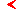mainscience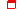this page only

# Period-doubling critical behavior

## C - Cycle of the RG equationsalphabet

Description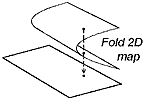Non-invertible 2D maps with fold singularity, flow systems of dimension 4 and more.

RG equations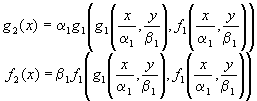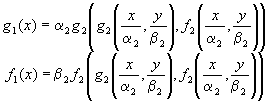Period-2 cycle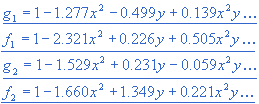The orbital scaling factors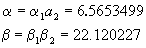Critical multipliers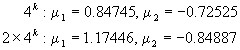Relevant eigenvalues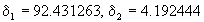Irrelevant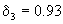(close to 1!)

Codimension
CoDim=2 (restr. 2)

Basic model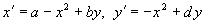at b=-0.6663:Scaling of the parameter plane with factors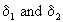Scaling coordinates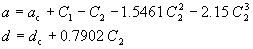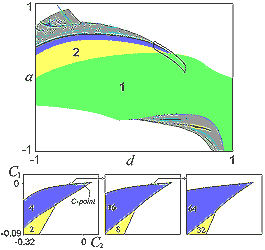show enlarged figure

Critical quasiattractor
An infinite set of stable cycles of period 4kmainsciencealphabetthis page only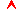top

Saratov group
of theoretical nonlinear
dynamics
Хостинг от uCoz